# AFCAT Exam Topic Based Important Sample Question Answer Set 2## AFCAT Exam Topic Based Important Sample Question Answer Set 2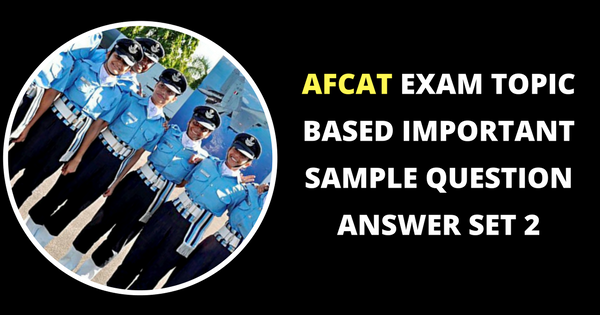1. There were 24 students in a class. One of them, who was 18 years old, left the class and his place was filled up by a new comer. If the average of the class was thereby lowered by 1 month, the age of new comer is- a. 14 years b. 15 years c. 16 years d. 17 years Solution. Let new comer age was x. Average age decreased by = 1 month = 1/12 years. No. of students in class * aged decreased = difference of replacement 24 * 1/12 = 18 – x 2 = 18 – x x = 16 years. Answer. c
 2. A bag contains 25 paise, 50 paise and 1 Rs coins. There are 220 coins in all and the total amount in the bag is Rs . 160. If there are thrice as many 1 Rs. Coins as there are 25 paise coins, then what is the number of 50 paise coins? a. 60 b. 40 c. 120 d. 80 Solution.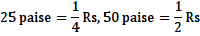Let the number of 25 paise coins = x Then number of 50 paise coin = 3x And let the number of 50 pasie coin = y Then,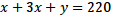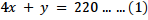Now,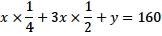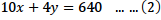Solving (1) and (2) we get x = 40 and y = 60 Answer. a
 3. The average weight of 5 men is increased by 2 Kg when one of the men whose weight is 60 Kg is replaced by a new man. The weight of the new man is a. 50 Kg b. 65 Kg c. 68 Kg d. 70 Kg Solution. (60+a+b+c+d)/5 = W ——(i) (n+a+b+c+d)/5 = W+2 —–(ii) (ii) – (i) n-60 = 10 n = 70 Kg Answer. d
 4. If a sum becomes double in 16 years, how many times will it be in 8 years? a. 1(1/2) times b. 1(1/3) times c. 1(3/4) times d. 1(1/4) times Solution. Let the sum be S Sum in 16 yrs —— 2S increase is 100 % so for 8 yrs  increase will be 50 % Answer. a
 5. Srinivasan invests two equal amounts in two banks giving 8% and 12% rate of interest respectively. At the end of year the interest earned is Rs 1500. Find the sum invested in each. a. 8500 b. 15000 c. 7500 d. 17000 Solution. One must understand that Rs 1500 is the total interest earned on two unknown but equal amounts at 8% and 12%. Let ‘y’ be the amounts then, adding the interestsAnswer. c
 6. In a mixture of 60 litres, the ratio of milk and water is 2:1. What amount of water must be added to make the ratio of milk and water as 1:2 ? a. 42 Litres b. 56 Litres c. 60 Litres d. 77 Litres Solution. Water in initial mixture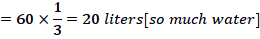Milk in the initial mixture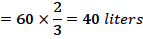Let ‘x’ be the water that is added later to change the ratio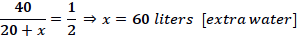Answer. c
 7. The salary of A & B together amounts to Rs.2000. A spends 95% of his salary and B 85% of his salary. If their savings are same what is the salary of A? a. 750 b. 1250 c. 1500 d. 1600 Solution. (5/100) A = (15/100) B A = 3B A + B = 2000 4B = 2000 => B = 500 A = 1500 Answer. c
 8. How much time will it take for an amount of Rs.450 to gain Rs.81 as interest, if rate of interest is 4.5% p.a on simple interest? a. 4.5 years b. 3.5 years c. 5 years d. 4 years Solution.  PTR/100 Time = (100×81/ 450×4.5)years => 4 years. Answer. d
 9. A loss of 19% on a shirt gets converted into a profit of 17% when the selling price is increased by Rs 162. What is the cost price of the shirt? a. Rs 540 b. Rs 450 c. Rs 600 d. Rs 360 Solution. A change from 19% loss to 17% gain means a change of 36%   36% = 162   1% = 162/36   100% = 162/36 × 100 = 450 Rs Answer. b
 10. If the selling price of an article is 4/3 of its cost price, the profit in transaction is a. 16.75% b. 20.50% c. 25.50% d. 33.33% Solution. Lets assume CP as Rs.300/- So SP will be 300 x (4/3) = Rs.400/- Profit = SP-CP= Rs.100/- ie if SP is 4/3=(1.3333) then CP will be 1 and profit will be 1.33333–1=0.3333 1/3 = 0.3333 Answer. d
 11. If a/(a+b) = 17/23, what is (a+b)/(a-b) equal to? a. 13/7 b. 23/11 c. 14/5 d. 25/9 Solution.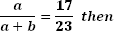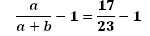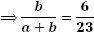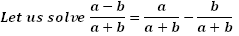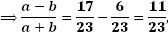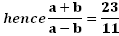Answer. b
 12. A sum of money is to be distributed among P, Q and R in the ratio of 6:19:7. If R gives Rs 200 from his share to Q. the ratio of P, Q and R becomes 3: 10:3, what is the total sum? a. Rs 3200 b. Rs 12800 c. Rs 6400 d. Data inadequate Solution. Let the 6x, 19x and 7x be the money distributed among, P, Q and R respectively,   Hence, 6x + 19x + 7x = 32 x is the total amount of money   After R gives Rs 200 to Q, there ratio become 3x:10x:3x = 6x:20x:6x,   if we compare this ratio to the above ration we will see a change from 7x to 6x and 19x to 20x,   Hence there difference i.e x = 200,   Therefore the total amount of money = 32x = 32× 200 = 6400 Rs Answer. c
 13. 75 pupils from a school appeared for an examination and 80% of them passed. Another school entered 10 more pupils than the first school and five pupils less than the first school passed. The pass % of in the second school was a. 75% b. 84% c. 72% d. 64.7% Solution. Number of students who passed in the first school 75 x 0.8 = 60 Number of students who appeared for exam in the second case = 75 + 10 = 85. Number of students who passed from the second school is 5 less than the first school. 60 – 5= 55 Hence, the passing percentage of the second schoolAnswer. d
 14. Find the average of all the numbers between 6 and 34 which are divisible by five. a. 18 b. 20 c. 20 d. 30 Solution.Answer. b
 15.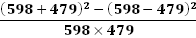a. 2 b. 6 c. 4 d. 8 Answer. c
 16.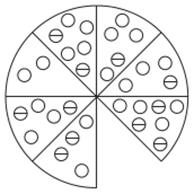Which section is missing?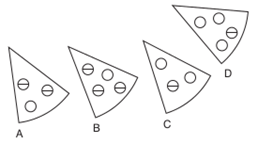a. A b. B c. C d. D Solution. C: added together, opposite segments contain six empty circles and three with a stripe Answer. c
 17. Which is the odd one out?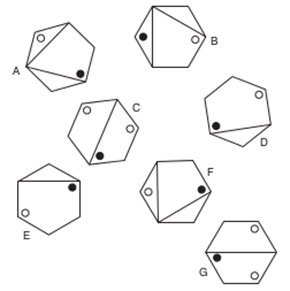a. A b. B c. C d. D Solution. B: A is the same as F rotated, C is the same as G and E is the same as D Answer. b
 18.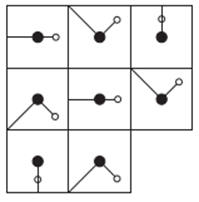Which is the missing tile?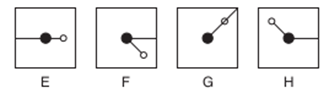a. E b. F c. G d. H Solution. E: looking across, the long line is moving 45° clockwise at each stage and the small line 45° anti clockwise. Looking down, the reverse is happening. Answer. a
 19.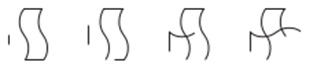What comes next?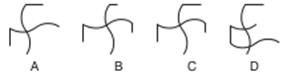a. A b. B c. C d. D Solution. C: the horizontal figure is disappearing by half a side at each stage and the vertical figure is appearing by half aside at each stage Answer. c
 20. Which is the odd one out?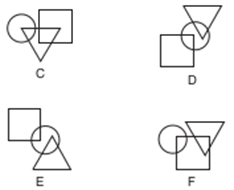a. C b. D c. E d. F Solution. E: in all the others the triangle is upside down Answer. c